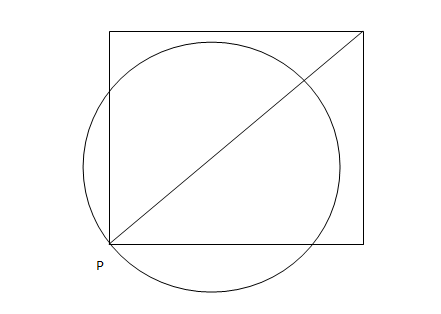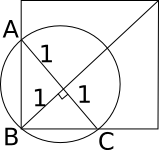### CAT 2006 Question Paper Question 2

Instructions

A punching machine is used to punch a circular hole of diameter two Units from a square sheet of aluminium of width 2 units, as shown below. The hole is punched such that the circular hole touches one corner P of the square sheet and the diameter of the hole originating at P is in line with a diagonal of the square:Question 2

# Find the area of the part of the circle (round punch) falling outside the square sheet.

SolutionThe area of triangle ABC is 1/2 * $$\sqrt2$$ * $$\sqrt2$$ = 1
Area of semi-circle ABC = $$\pi/2$$
So, area of circle outside the square = $$\pi/2$$ - 1 = ($$\pi$$ -2)/2